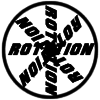#### You may also like### 8 Methods for Three by One

This problem in geometry has been solved in no less than EIGHT ways by a pair of students. How would you solve it? How many of their solutions can you follow? How are they the same or different? Which do you like best?### Rots and Refs

Follow hints using a little coordinate geometry, plane geometry and trig to see how matrices are used to work on transformations of the plane.### Reflect Again

Follow hints to investigate the matrix which gives a reflection of the plane in the line y=tanx. Show that the combination of two reflections in intersecting lines is a rotation.

# Nine Eigen

### Why do this problem?

This problem asks a series of questions designed to provoke students' thinking about matrices which leave vectors fixed, and the properties that such matrices and vectors would have.

### Possible approach

It may be worthwhile to start with some preliminary work about matrices in three dimensions. Students could find some examples of $3 \times 3$ matrices which represent simple rotations and reflections, which could be used in answering the problem.

The questions divide neatly into three sections - questions 1-3, 4-6 and 7-9. Students could tackle these questions in those three sections, perhaps working with a partner, and feed back ideas to the rest of the class after each section is answered.

For each section of questions, ask students to think about what they are being asked to do, use their intuition to make any initial comments, then think about the geometry of the situation and finally use some examples to support their thoughts algebraically.

### Key questions

What can you say about a rotation that leaves the direction of a vector unchanged?
What can you say about a reflection that leaves the direction of a vector unchanged?

### Possible extension

Fix Me or Crush Me investigates matrices which fix certain vectors and vectors which are fixed by certain matrices.

### Possible support

Matrix Meaning provides some simpler questions about the possible effects of different types of $3 \times 3$ matrices.